Two boats approach a light house in mid-sea from opposite directions.
Question:

Two boats approach a light house in mid-sea from opposite directions. The angles of elevation of the top of the light house from two boats are 30° and 45° respectively. If the distance between two boats is 100 m, find the height of the light house.

Solution:

Let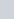be the height of light house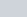. Angle of elevation of the top of light house from two boats are 30° and 45°. Let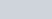,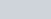and it is given that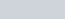m. So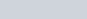. And,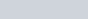Here we have to find height of light house.

The corresponding figure is as followsSo we use trigonometric ratios.

In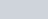,

$\Rightarrow \quad \tan 45^{\circ}=\frac{B D}{B C}$

$\Rightarrow \quad 1=\frac{h}{x}$

$\Rightarrow \quad x=h$

Again in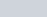$\Rightarrow \quad \tan 30^{\circ}=\frac{D B}{A B}$

$\Rightarrow \quad \frac{1}{\sqrt{3}}=\frac{h}{100-x}$

$\Rightarrow \quad \sqrt{3} h=100-x$

$\Rightarrow \quad \sqrt{3 h}=100-h$

$\Rightarrow(\sqrt{3}+1) h=100$

$\Rightarrow \quad h=\frac{100}{\sqrt{3}+1} \times \frac{\sqrt{3}-1}{\sqrt{3}-1}$

$\Rightarrow \quad h=50(\sqrt{3}-1)$

Hence the height of light house is $50(\sqrt{3}-1) \mathrm{m}$.